StatLect

# Model misspecification

Model misspecification happens when the set of probability distributions considered by the statistician does not include the distribution that generated the observed data.## Statistical model

To properly understand misspecification, we first need to define statistical models (e.g., McCullagh 2002).

Definition A statistical model is a set of probability distributions that, according to the statistician's judgement, should contain the probability distribution from which the data have been drawn.

Let us illustrate the definition with some examples.

Example The data consists of height measurements forindividuals drawn at random from a population. Formally, the measurements can be seen as the realizations ofrandom variables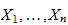. These variables could have any probability distribution. If the statistician assumes that they are normally distributed, then she is formulating a statistical model. She is placing a restriction on the set of probability distributions from which the data could have been drawn.

Example In the previous example, there could be some form of dependence among the draws. If the statistician assumes that the draws are statistically independent, then she is putting another restriction on their joint distribution. In other words, she is adding an assumption to the statistical model.

Example The statistician measures the weights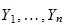of the sameindividuals. Then, she analyzes the relationship between height and weight by using the regression equation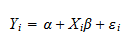whereandare regression coefficients andis an independently and identically distributed error term. This is a statistical model: the statistician is putting a restriction on the set of joint distributions that could have generated the observations; she is excluding all the joint distributions for which the errorsare not independently and identically distributed.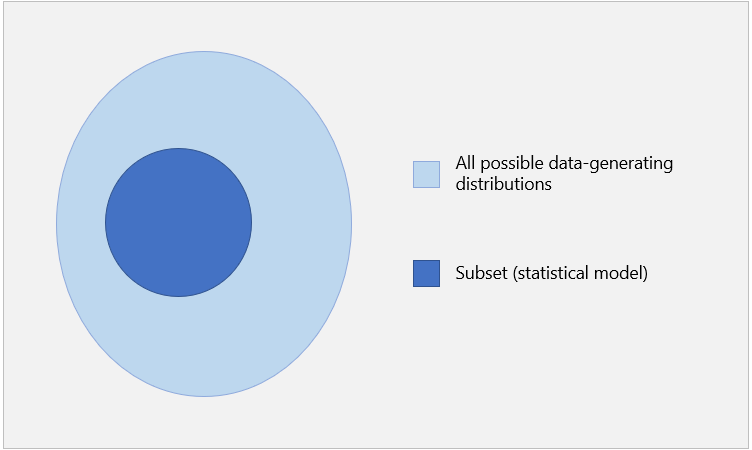## Misspecified model

Thus, a model is a set of probability distributions. If the data-generating distribution does not belong to the set, then the model is misspecified.

As we build a model by making assumptions, a model is misspecified when at least one of our assumptions is wrong.

Example Theindividuals in the previous examples belong to two ethnic groups characterized by different genetic traits. In particular, there is a marked difference between the average heights of the members of the two groups. The true data-generating distribution is bimodal and does not belong to the set of normal distributions. Hence, the previous model is misspecified.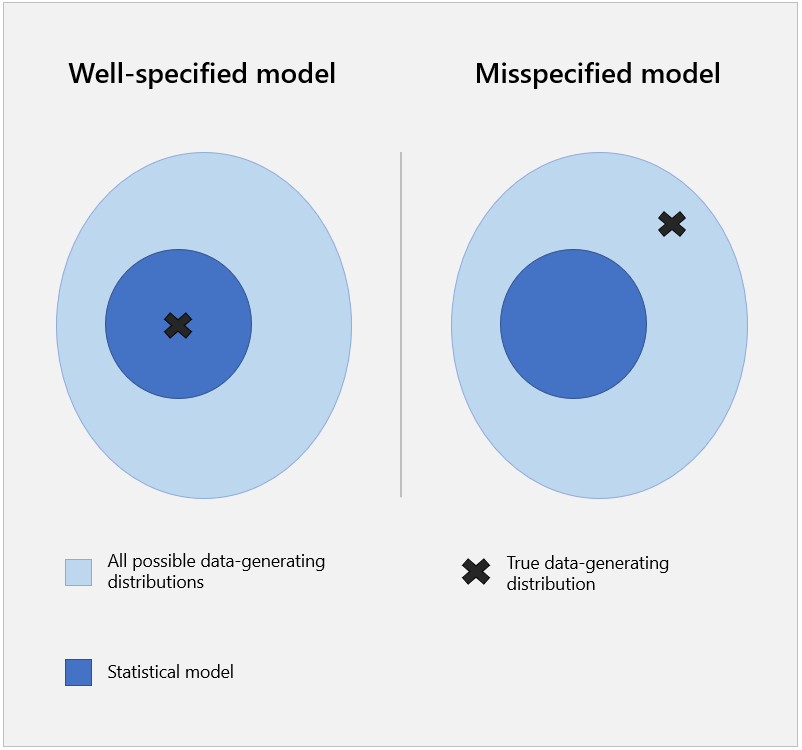## Consequences of misspecification

The assumptions that form the statistical model are used to derive the properties of the estimators of the model parameters and the distribution of any test statistic used to conduct hypothesis tests about the data-generating distribution.

As a consequence, in a misspecified model, the behavior of estimators and test statistics may be different from the one that we would expect on the basis of the assumptions.

Example In a test of hypothesis, even if the main assumption being tested is true, we may reject it too often because another minor (or technical assumptions) is wrong. See the section on the interpretation of rejection in the lecture on the null hypothesis. A related problem is that we may end up accepting the alternative hypothesis (after rejecting the null) even if both the null and the alternative are wrong. See the lecture on the alternative hypothesis.

Example If we assume that the errors of a linear regression are homoskedastic (i.e., they all have the same variance), then the standard errors of the coefficient estimates have a simple expression. However, if the model is misspecified because the homoskedasticity assumption is wrong, then the standard errors are inconsistent. Fortunately, we can drop the assumption of homoskedasticity and use heteroskedasticity-robust estimators.

Example If we assume that the observations in a sample are independently, identically and normally distributed, then their sample mean has a normal distribution. However, if the model is misspecified because the observations are not normal, then the distribution of the sample mean may not be normal, especially in small samples.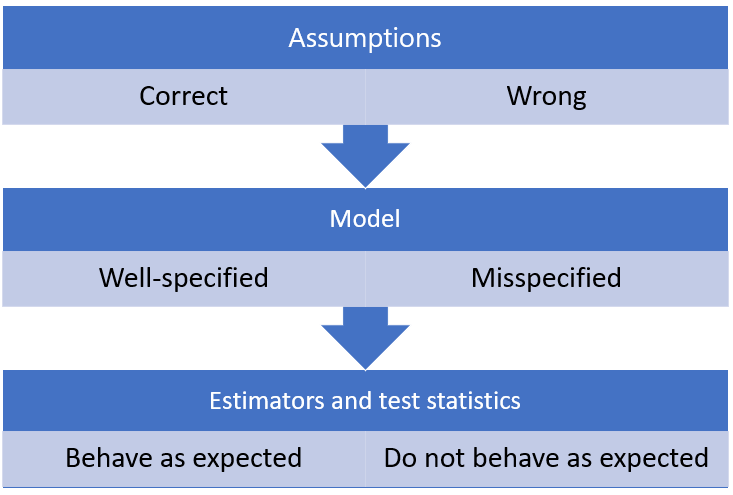## Incorrect definitions

Sadly, there is a lot of confusion about misspecification.

In particular, you will find many sources that do not provide a formal definition of misspecification and provide lists of examples. These often include some problems that may affect regression models such as:

• omission of relevant variables;

• inclusion of irrelevant variables;

• wrong functional form.

While some of these problems may in certain cases be related to misspecification, their presence does not necessarily imply that the model is misspecified. Let us see why.

## Misspecified linear regression

When we write a regression equation of the formwe are not placing any restriction on the joint distribution ofand.

In other words, we are not making any assumption. We are just defining a new variableas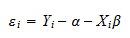At this stage,is not even well-defined, because the coefficientsandare left unspecified.

### Included and excluded variables

When we write the regression equation and we define the error term, we select the variables to include in the equation.

In the above equation we have included only one regressor (), but we could have made different choices.

For example, we could have included a second variable:whereis another regression coefficient.

The inclusion/exclusion of a variable is not per se a cause of misspecification because:

• the decision only affects the way in which the erroris defined; it determines which joint distribution we are analyzing (in the latter case, the joint distribution of,and);

• no restriction whatsoever is imposed on the distribution of the variables being analyzed.

### Assumptions about the error term

In a second stage, we make some assumptions about the error term.

These assumptions serve two purposes:

1. to uniquely pin down the regression coefficientsand(even if the latter remain unknown to the statistician);

2. to place restrictions on the joint distribution of the variables included in the regression.

### Identification assumptions

The assumptions in point 1 are called identification assumptions because they are needed to uniquely identify the regression coefficientsand.

Usually, the identification assumptions are:

• the error termis orthogonal to the regressors;

• the regressors cannot be perfectly multicollinear.

Mathematically, these two assumptions are equivalent to saying that the true regression coefficients are the coefficients of the orthogonal projection of the dependent variable on the regressors (e.g., Kachapova and Kachapov 2010).

Thanks to the identification assumptions, the regression model is well-defined. But the identification assumptions do not place any restriction on the joint distribution of the variables. They simply allow us to provide a precise mathematical definition of the regression coefficients, which would otherwise be undefined objects.

It follows from these considerations that also the identification assumptions cannot be a cause of misspecification.

### Assumptions that can cause misspecification

Any other assumptions (beyond those made in the selection of regressors and those needed for identification) can cause misspecification.

Some commonly made ones are:

These are true restrictions on the data-generating distribution. If the latter does not satisfy these restrictions, then the regression model is misspecified.

### Problems often mislabeled as misspecification

As we said above, several important problems are often incorrectly considered misspecification problems.

For example:

• Omitted variable bias: due to the exclusion of some regressors, the regression coefficients do not have a causal interpretation (e.g., Angrist and Pischke 2009).

• Irrelevant regressors: due to the presence of many regressors that are unlikely to be correlated with the dependent variable, the OLS estimators of the regression coefficients have high variance.

• Inappropriate functional form: by formulating a different regression model (where the variables are transformed through nonlinear functions), the variance of the error terms would decrease considerably.

When these problems are present, there may exist a better regression model than the one we have chosen. For example, a different model may be more interpretable, easier to estimate precisely, or it may produce better forecasts.

But the fact that we can improve our model in some respect does not mean that our model is misspecified. In other words, all of the assumptions we have made may still be satisfied by the data-generating distribution.

Spanos (2011) provides one of the most articulated discussions about this point, where he clearly distinguishes between:

• statistical adequacy (i.e., the lack of misspecification);

• substantive adequacy (i.e., the lack of significant opportunities to improve the model).

### Caveat

While the presentation above should be consistent with modern and rigorous treatments of statistical models and linear regressions, many authors continue to include problems such as the omitted variable bias under the misspecification umbrella.

Moreover, highly-cited scientific articles explicitly label the existence of better functional forms as misspecification (see, e.g., Ramsey's 1969 RESET test).

If you are a student and you need to take a test that may include questions about misspecification, check what definition of misspecification is used by your instructor or by the institution that administers your exams.## References

Angrist, J. D. and Pischke, J. S. (2009). Mostly harmless econometrics: An empiricist's companion. Princeton University Press.

Farida Kachapova and Ilias Kachapov (2010). Orthogonal Projection in Teaching Regression and Financial Mathematics, Journal of Statistics Education, Volume 18, Number 1, 1-18.

McCullagh, P. (2002). What is a statistical model? The Annals of Statistics, 30(5), pp.1225-1310.

Ramsey, J. B. (1969). Tests for Specification Errors in Classical Linear Least Squares Regression Analysis. Journal of the Royal Statistical Society, Series B. 31 (2): 350-371.

Spanos, A. (2011). Foundational issues in statistical modeling: Statistical model specification and validation. RMM, Vol. 2, 2011, 146--178, Special Topic.

## Keep reading the glossary

Previous entry: Mean squared error

Next entry: Multinomial coefficient